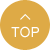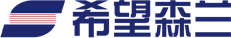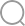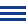| EN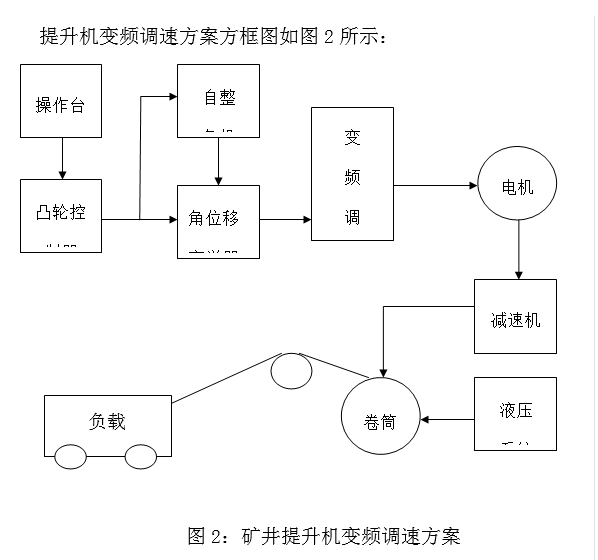（1）变频系统操作时和原来工频系统在设备状态上有所不同，在提升机控制台上有个凸轮控制器，（其作用是利用控制器的多接点当作提升机的档位控制接触器，调节转子电阻的阻值大小，对应低高速档。）除开油路部分的控制触点以外的所有接点弹簧全部已被去掉，这样有利于在使用角位控制时候减少操作阻力。

（2）再将绕线式电机的转子绕组全部短接。

（3）利用原来的凸轮控制器，在凸轮控制器的后方轴上安装自整角机，与凸轮控制器的中心轴同心，这样利用原来上升和下放的操作模式。对应凸轮控制器的正负角度，分别角度是108°和-108°，自整角机把这个角位信号以脉冲形式送给角位变送器，角位变送器再根据正负角度对应输出+108°与-108°时的+10V和-10V的模拟电压信号，变频器接收±10V作为正反转运行信号。

（4）利用原凸轮控制器控制制动油路系统，在凸轮控制器上中心点到相对应两边第一个触头须使用。度过两边的触点后，随着操纵杆的推动平滑地过渡到108°。在操纵杆未打到油路系统触点时，自整角机已经识别到角位的变化，对应角位变送器就会有正负电压的输出，因为在油路系统未打开之前变频器是有很低的频率输出（0～0.3Hz）。油路未打开刹车系统就处于制动状态，通过变频器内部的逻辑单元、算术单元和比较器等相关功能进行设置，过渡了两个接触点的清零位置，使得在操纵杆在过油路触点时候变频器在0.2Hz以下运行，得到非常好的控制效果。

 F0-01=7 F0-02=1 F1-00=7 F1-01=3 F1-18=1.0 F1-25=1 F2-01=2 F2-04=90 F4-01=16 F4-08=0 F5-00=49 F5-01=14 F5-03=5 F6-00=6 F6-15=1 F6-16=101.1 FE-00=10 FE-01=28 FE-02=11 FE-03=25 FE-05=44

 F6-15=1 F6-16=101.1 FE-00=10 FE-01=28 FE-02=11 FE-03=25 FE-05=44 FE-06=10 FE-07=29 FE-08=1 FE-09=0 FE-12=1 FE-13=50 FE-14=0 FE-16=14 FE-18=7 FE-44=29 FE-46=8 FE-48=27 FE-49=31 FE-50=3 FE-51=70 FE-52=10 FE-53=28 FE-54=1 FE-56=10 FE-57=28 FE-72=21 FE-73=22 FE-74=48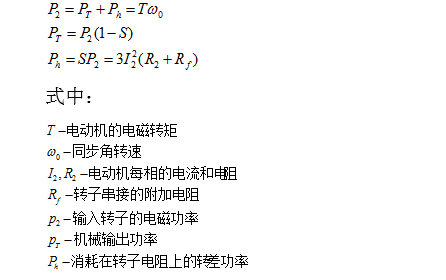5、结束语